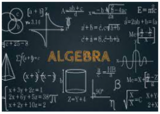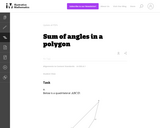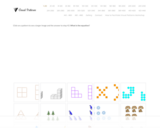Updating search results...

# 4 Results

View
Selected filters:
• UT.MATH.II.A.CED.1Conditional Remix & Share Permitted
CC BY-NC
Rating
0.0 stars

This unit was created as an introductory/review unit for Algebra II to allow for a review of main concepts needed to progress in Algebra II. However, it could also be used in Algebra I to introduce for the first time real numbers;&nbsp;number operations;&nbsp;and equations, expressions, and inequalities.

Subject:
Secondary Mathematics
Material Type:
Homework/Assignment
Lesson
Unit of Study
Author:
Emily
02/22/2022Unrestricted Use
CC BY
Rating
0.0 stars

This problem provides students with an opportunity to discover algebraic structure in a geometric context. More specifically, the student will need to divide up the given polygons into triangles and then use the fact that the sum of the angles in each triangle is 180_.

Subject:
Mathematics
Secondary Mathematics
Material Type:
Activity/Lab
Provider:
Illustrative Mathematics
Provider Set:
Illustrative Mathematics
Author:
Illustrative Mathematics
01/21/2013Unrestricted Use
CC BY
Rating
0.0 stars

Multiple sets of visual patterns that follow a variety of patterns such as linear, exponential, quadratic, etc. Can be used to have students figure out what the next set of shapes will look like, write equations to describe the pattern, and predict how many shapes will be the 43rd set or beyond.

Subject:
Mathematics
Secondary Mathematics
Material Type:
Diagram/Illustration
Author:
Fawn Nguyen